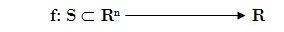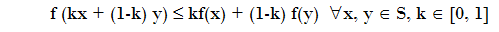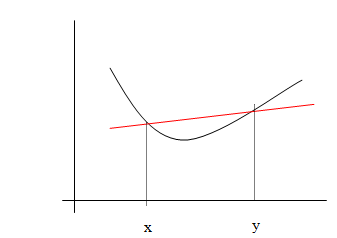Convex Function

LetsWith S convex set.
We say that f is a Convex Function ifThe intuitive idea is that the graph of the function between x and y lies below the secant.Note 1: If we substitute less than or equal to greater than or equal, is the definition of concave function.
Note 2: If we substitute less than or equal to less strict is the definition of strictly convex function.
Note3: If replacing less than or equal to less strict, we strictly concave function definition.